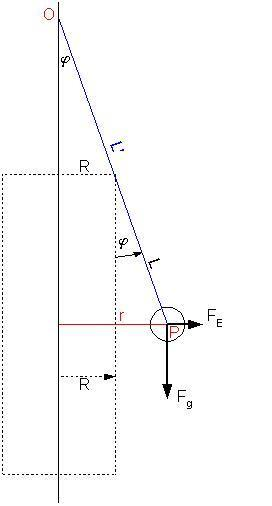# Charged ball hanging from the side of charged cylinder, great complication.

## Homework Statement

We have a charged ball with charge e and mass m hanging from the side of the charged cylinder with radius R and surface charge density of $\sigma$.
The string to which the ball is attached is of length L

Find a relation between charge e on the ball and the angle $\varphi$ between cylinder and string.

http://www.shrani.si/?2J/AQ/40Q2N2jg/cyllinder.png

## Homework Equations

Gauss' law for calculating electric field where ball is.

## The Attempt at a Solution

Gauss law for electric field around cylinder gives us:$E=\frac{\lambda}{2\pi \cdot x\cdot \epsilon_0}$

Considering linear density $\lambda$ equals $\sigma$2PI*R (R is radius of cylinder), we get the expression:

$E=\frac{\sigma R}{x \epsilon_0}$

$\sigma$ is surface charge density.

where x equals distance from centre of the cylinder to ball position, meaning $x = R + Sin[\phi]\cdot L$

After drawing a free body diagram and eliminating the force of string, we have the electric force and force of gravity left.

Electric force thus equals: $F_E=\frac{e\sigma R}{\epsilon_0 (R+Sin[\phi] L)}$

We get the followting expression:$Tan[\phi] = \frac{e\sigma R}{\epsilon_0 m g (R+Sin[\phi] L)}$

Trying to solve this for phi is pretty painful, even Mathematica can't really properly do it (even for substituting Tan with Sin for small angles)
Is there any other way for showing a relation between charge and angle?

Any help would be greatly appreciated.

Last edited by a moderator:

gneill
Mentor
The cylinder has the same electric field as a line of charge with the same linear charge density, so how about solving the problem as though the string were attached to the line of charge, then superimposing the cylinder afterwards to determine the shorter 'L' that corresponds to the cylinder case?

In the figure, the string length L' for the line-of-charge situation is the length OP. When the cylinder is superimposed, its 'new' length L can be found with a bit of trig.#### Attachments

If I understand you correctly, the main point is substituting x with something simpler, so we lose the transcendental identity, since everything else is pretty much the same.
So I went with that but unfortunately, everything I got using similar triangles and trig functions was something even more complex:(

For the new L I got $L=\frac{Sin[\phi] L^'}{R+Sin[\phi]}$

And using that with the R to get the distance doesn't simplify at all:( I'm sure I'm still missing something here hehe.

ehild
Homework Helper
The relation between e and phi was the question. Express e in terms of phi and the other parameters.

ehild

Maybe I've worded the question a bit wrong, PHI needs to be expressed in terms of charge and other parameters, sorry for confusion.

ehild
Homework Helper
You can express both the tangent and the sine of phi in terms of tan(phi/2) but it would be a fourth-order equation. Ask your teacher to show how he would solve it.

ehild